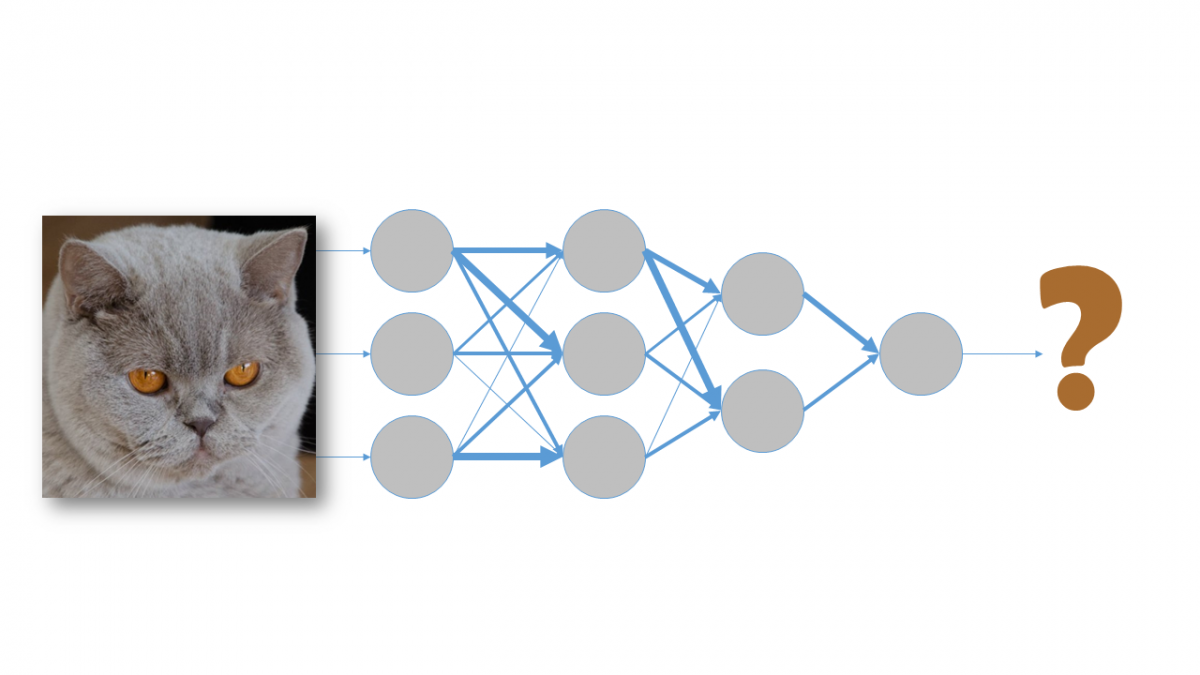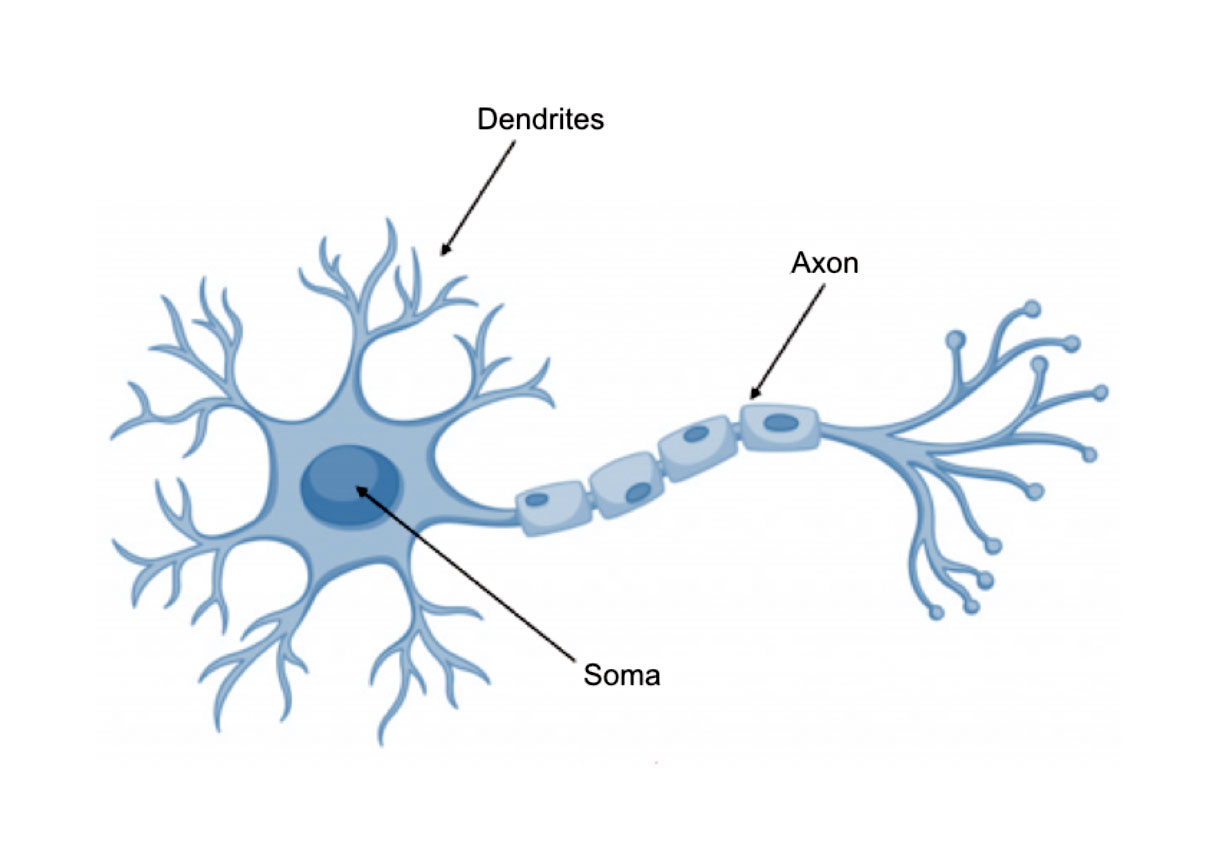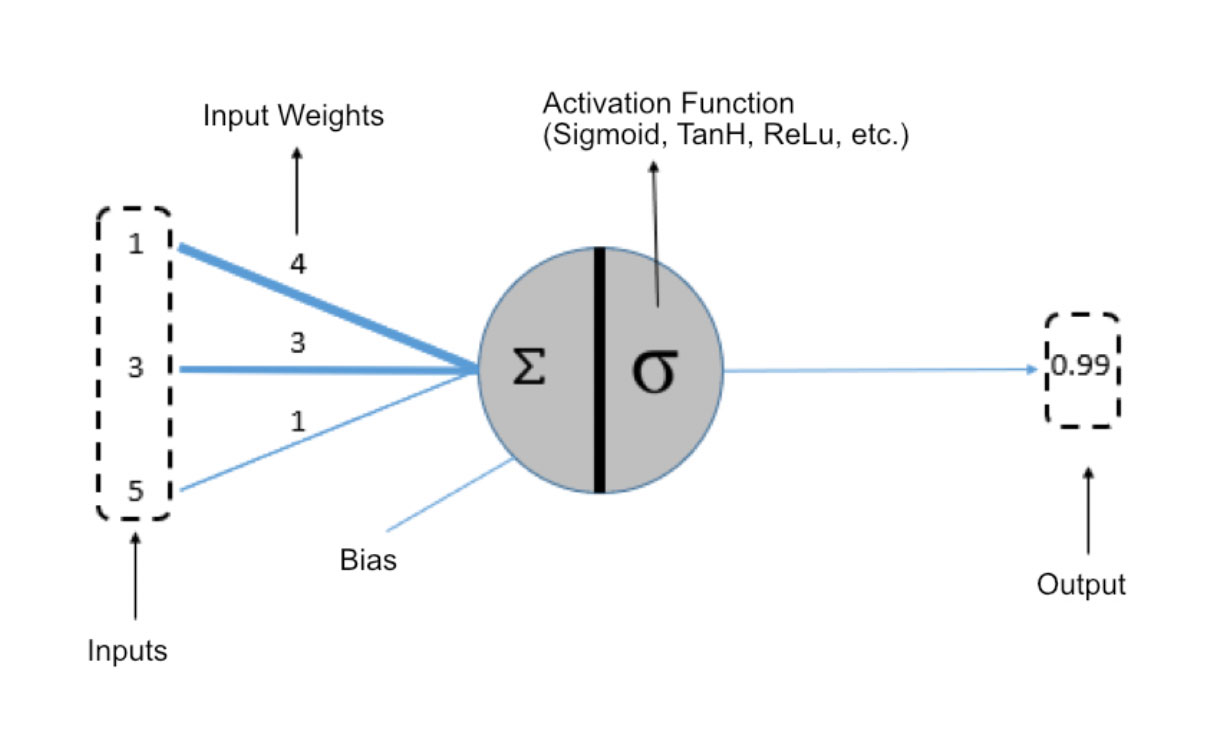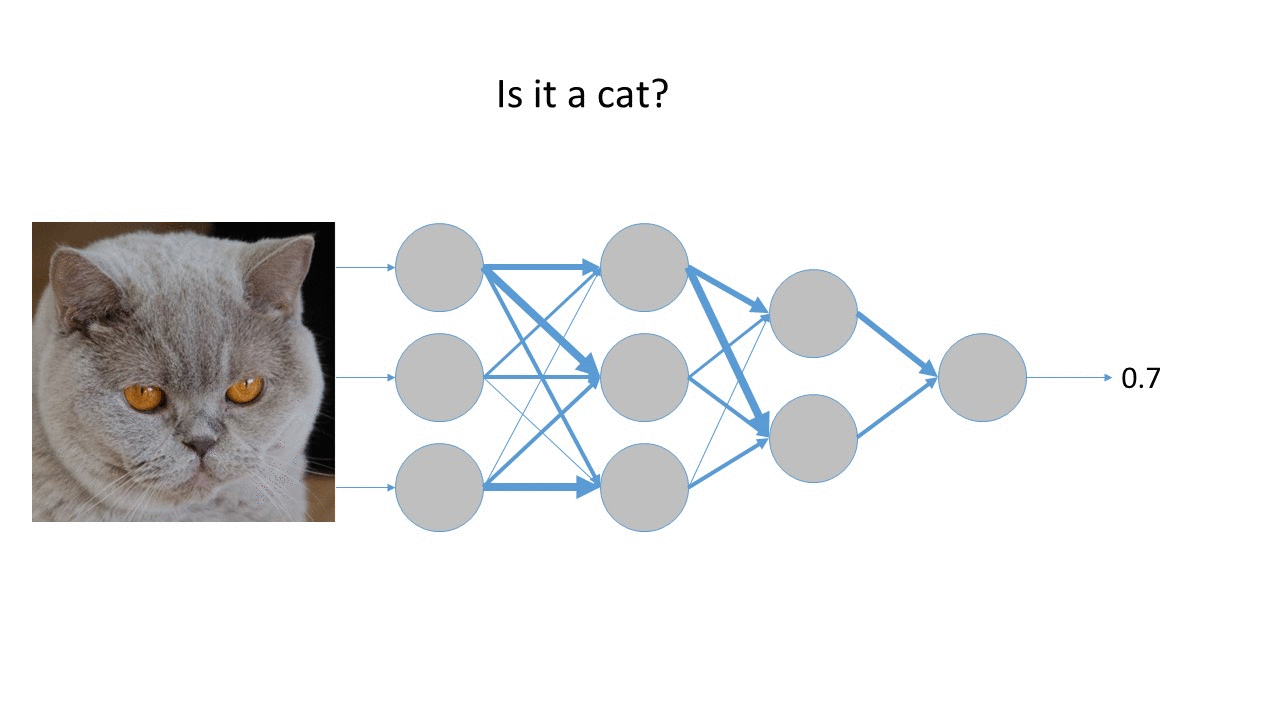Skip to main content

# Artificial Neural Networks: What they are and how they’re trained – Part IIn our earlier blog post about Machine Learning, we touched upon neural networks. In this post, we will explain what a neural network is and how it works.

Artificial neural networks are based on how networks of biological neurons work. The neurons that we all have in our brains are made up of dendrites, the soma and the axon. The job of the dendrites is to capture the nerve impulses emitted by other neurons. These impulses are processed in the soma and transmitted through the axon, which emits a nerve impulse to the adjacent neurons.## What is a neural network in Artificial Intelligence?

The following diagram represents an artificial neuron:In the case of artificial neurons, the sum of the inputs multiplied by their associated weights determines the “nerve impulse” that the neuron receives. This value is processed inside the cell by means of an activation function that returns a value that is sent as an output from the neuron.

Just as our brain is made up of interconnected neurons, an artificial neural network is made up of artificial neurons connected to each other and grouped into different levels that we call layers:

A layer is a set of neurons whose inputs come from an earlier layer (or input data in the case of the first layer) and whose outputs are the inputs for a later layer.

The following diagram shows a network with four layers:The neurons in the first layer receive as input the real data that feeds the neural network. For this reason, the first layer is known as the input layer. The output of the last layer is the visible result of the network, so the last layer is known as the output layer. The layers between the input layer and output layer are known as hidden layers because neither the input values nor output values are known to us.

Therefore, a neural network is always made up of an input layer, an output layer (if there is only one layer in the neural network, that layer is both the input layer and the output layer) and may contain zero or more hidden layers. The concept of Deep Learning was born out of the use of a large number of hidden layers in neural networks.

## How do you train a neural network?

Training a neural network consists of adjusting each of the input weights of all the neurons that are part of the neural network, so that the responses of the output layer match as closely as possible the data we know.

In the following animation, we can see a very simplified example of the neural network training process for, in this case, detecting a cat in an image. The thickness of each arrow represents the weight of that input in the neural network. As we can see, as the weights are adjusted, the error decreases.If we want the neural network to be able to generalise and identify cats in any image, it is important to use a large number of images during training – both images that are cats (labelled as 1) and images that are not cats (labelled as 0) – and to include as much variability as possible. In this way, the neural network will be able to adjust its parameters to correctly process as many images as possible, and it will therefore be able to extract accurately the characteristics that identify the presence of a cat in an image.

Now that we know what a neural network is and how they work, in our next blog post we’ll talk about the reasons why their use has become so widespread in recent years. Subscribe now and stay up to date with our latest posts on Artificial Intelligence.

is a Data Scientist and R&D Engineer at Xeridia## The Importance of Data Preprocessing in Artificial Intelligence: Data Cleansing